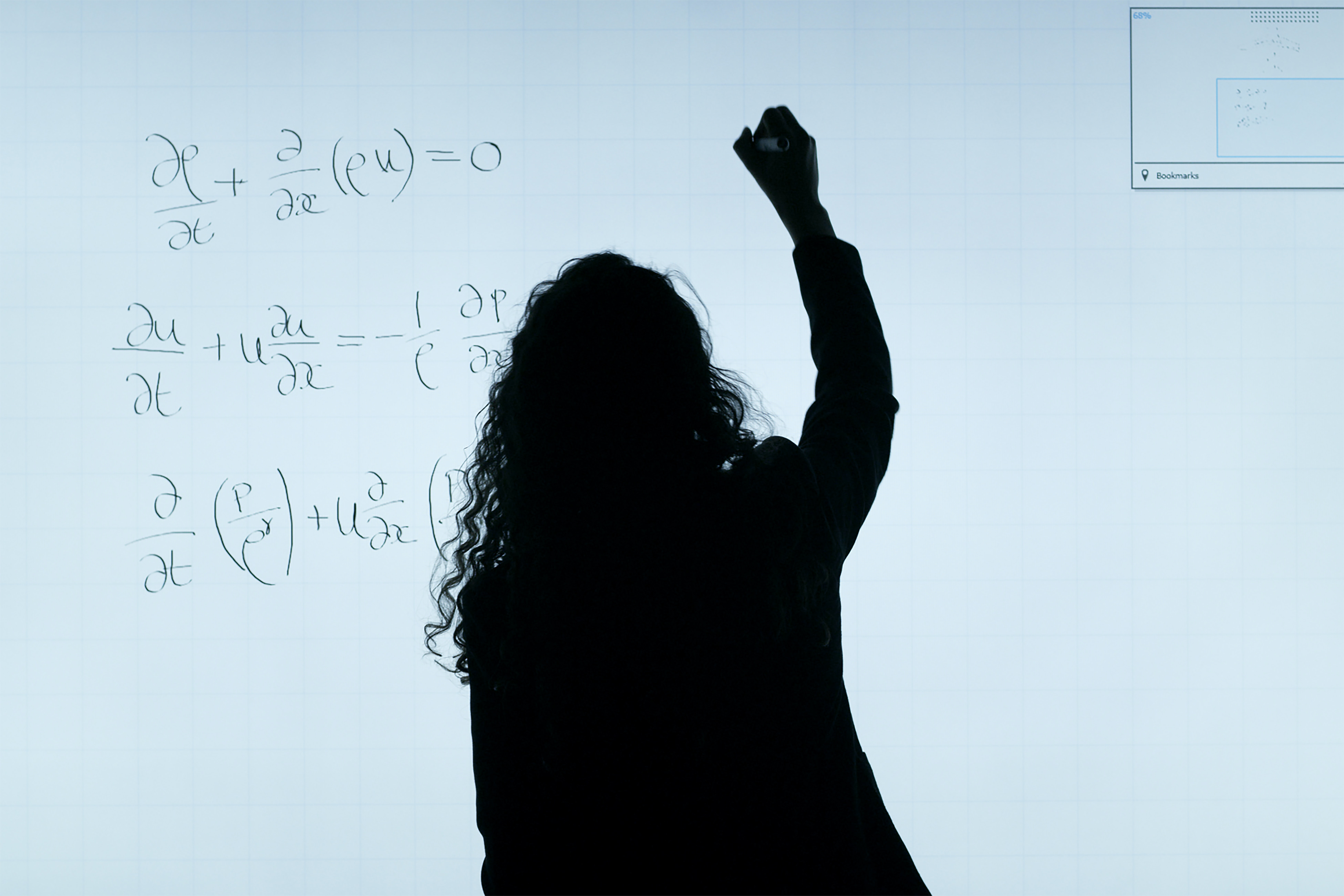# Pre-Calculus

Pre-Calculus builds on previous learning in Algebra 1, Geometry, and Algebra 2 or in Integrated Math 1, 2, and 3 to strengthen students’ mathematical reasoning and problem-solving.
Subject
Math
9-12Back to All CoursesIn this course you will

Explore exponential and logarithmic functions, trigonometric function and analysis, matrices and determinants, conic sections, and more

Strengthen mathematical reasoning and problem-solving